Hello, how are you? During this holiday, I'm treating myself to some well-deserved rest surrounded by greenery. Crickets and birds are singing, and kittens are playing right near me while I read and study a bit. I must admit, the last article about the first part of probability theory was quite challenging, but do not give up: let's make another small effort. Let's conclude the discussion about probability theory by talking about the probability function, but first, we have to answer the following important question:

"What is a function?"

Personally, I prefer to consider a function as a kind of arrow that connects an input value to a corresponding output value, just as shown in the image below:Keeping in mind this "definition" of a function, let's introduce the probability function, which is the "arrow" that connects an expected random variable value to the probability value that it will occur.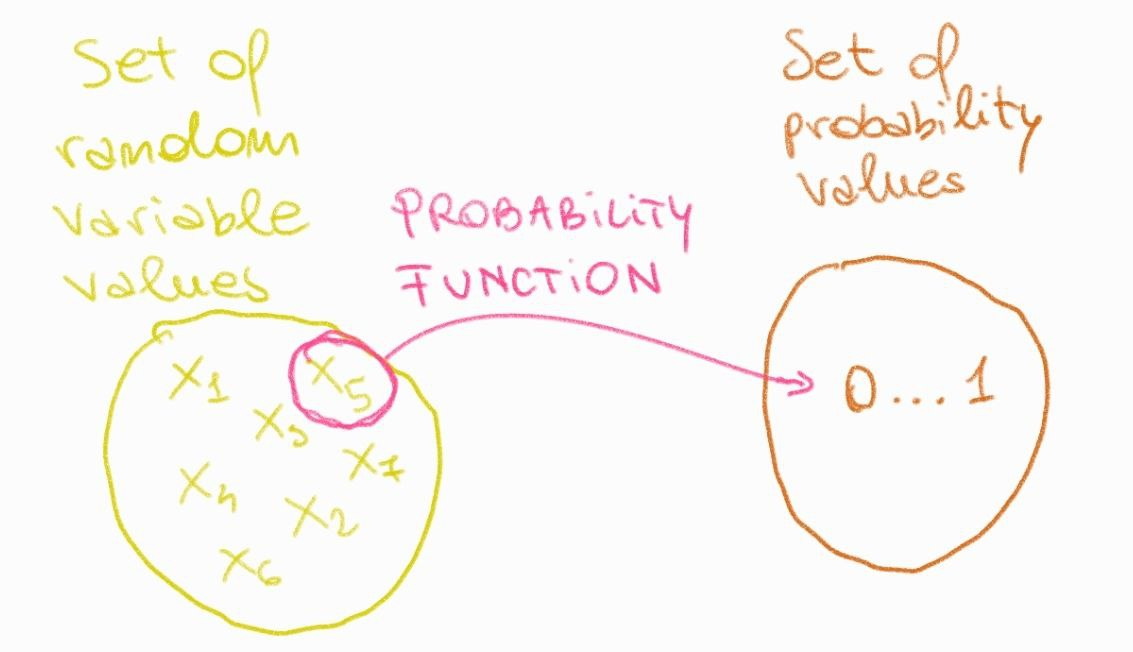Let's make some examples to clarify everything.

Example n.1:

Let's consider an unbiased six-sided die. What is the probability of getting a 6 as the result after rolling this die?

Defining the set of input values (die values from 1 to 6) and the set of output values (probability values from 0 to 1), the probability function is the "arrow" that connects the input value 6 to its probability of occurrence (output).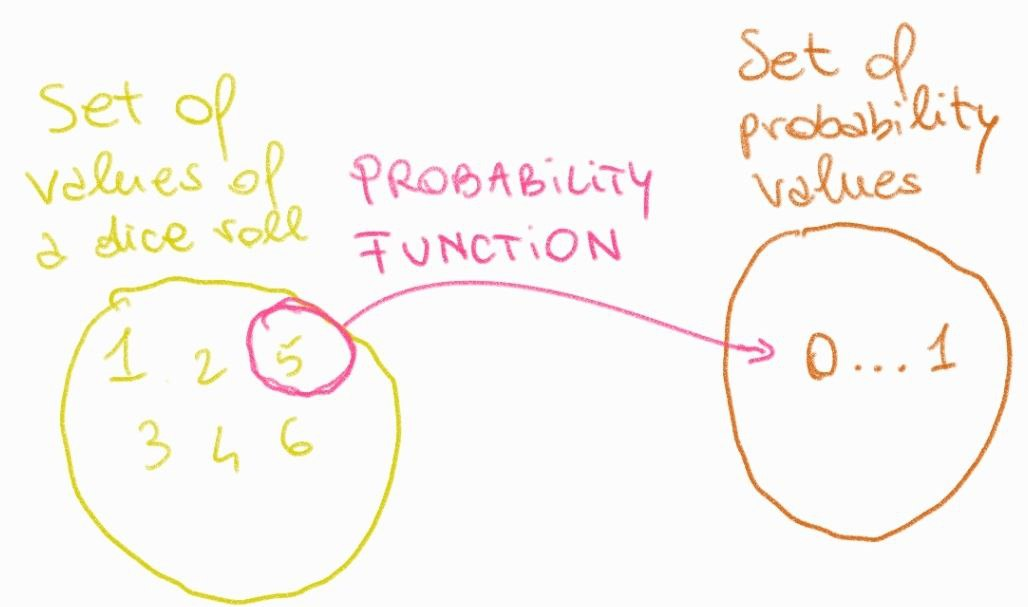By placing the input values on the x-axis and their respective probability values on the y-axis, we obtain a graph representing the distribution of probability values (see image below). In this case, the distribution is called "uniform" because each input value has the same output probability value.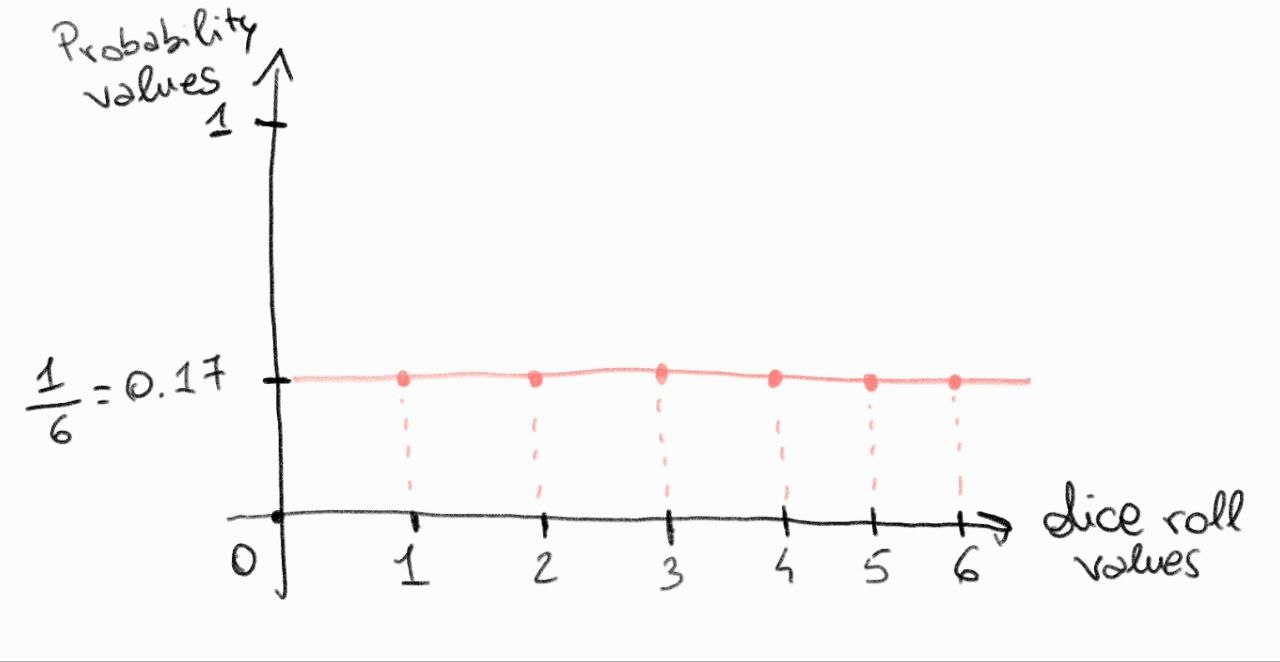Example n.2:

Now let's consider two unbiased six-sided dice. What is the probability that the total value is 9 after rolling both dice?

First of all, let's recall that after a roll, two dice can generate 36 different configurations since 6^2=36. Applying the classical method to calculate the probability of getting 9 as the sum of the rolls, we find that it is 0.11 (11%).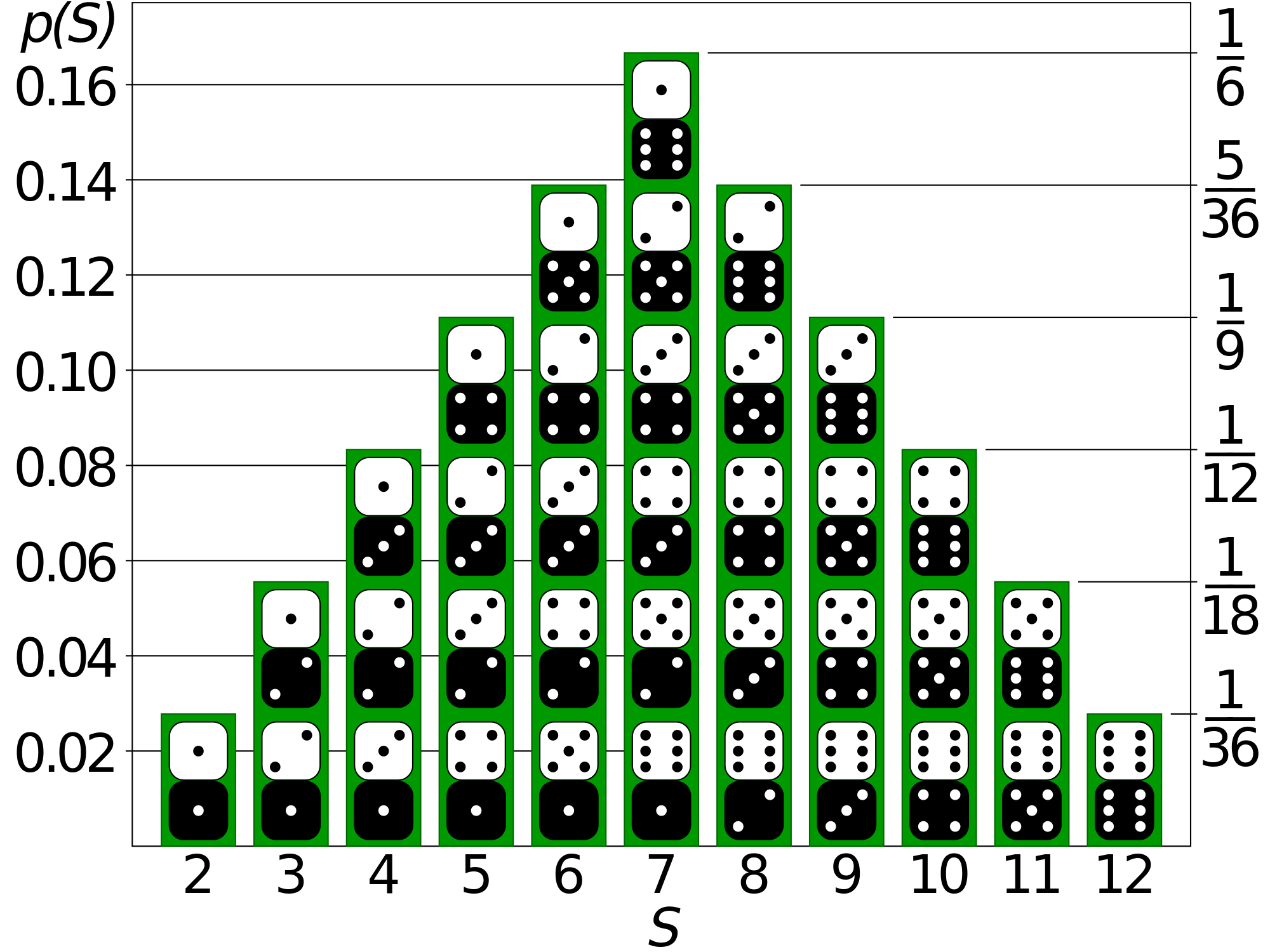It's important to emphasize that in example n.2, the distribution graph is not uniform, as each input has a different probability value.

A clarification is needed. The previously described probability function refers to discrete variables (NOT continuous) and is better known as the probability mass function (PMF). In the case of continuous variables, the probability function is called the probability density function (PDF).

Therefore, it's crucial to make this distinction:

• For discrete variables, the probability mass function allows us to calculate the probability of a specific input value occurring. The "arrow" connects an input value to an output value. Additionally, in this case, distribution graphs of probability values resemble bar graphs.
• For continuous variables, the probability density function, and the corresponding probability value for an input value, is determined by the integral of the function, which is the area under the curve in a range of values where the input value lies.

For now, the key concept to remember is:

The values of a random variable are "linked" to their respective probability values through a probability function. Also, by placing the probability values on the y-axis and the values of the random variable on the x-axis, it's possible to construct a graph called the "probability distribution."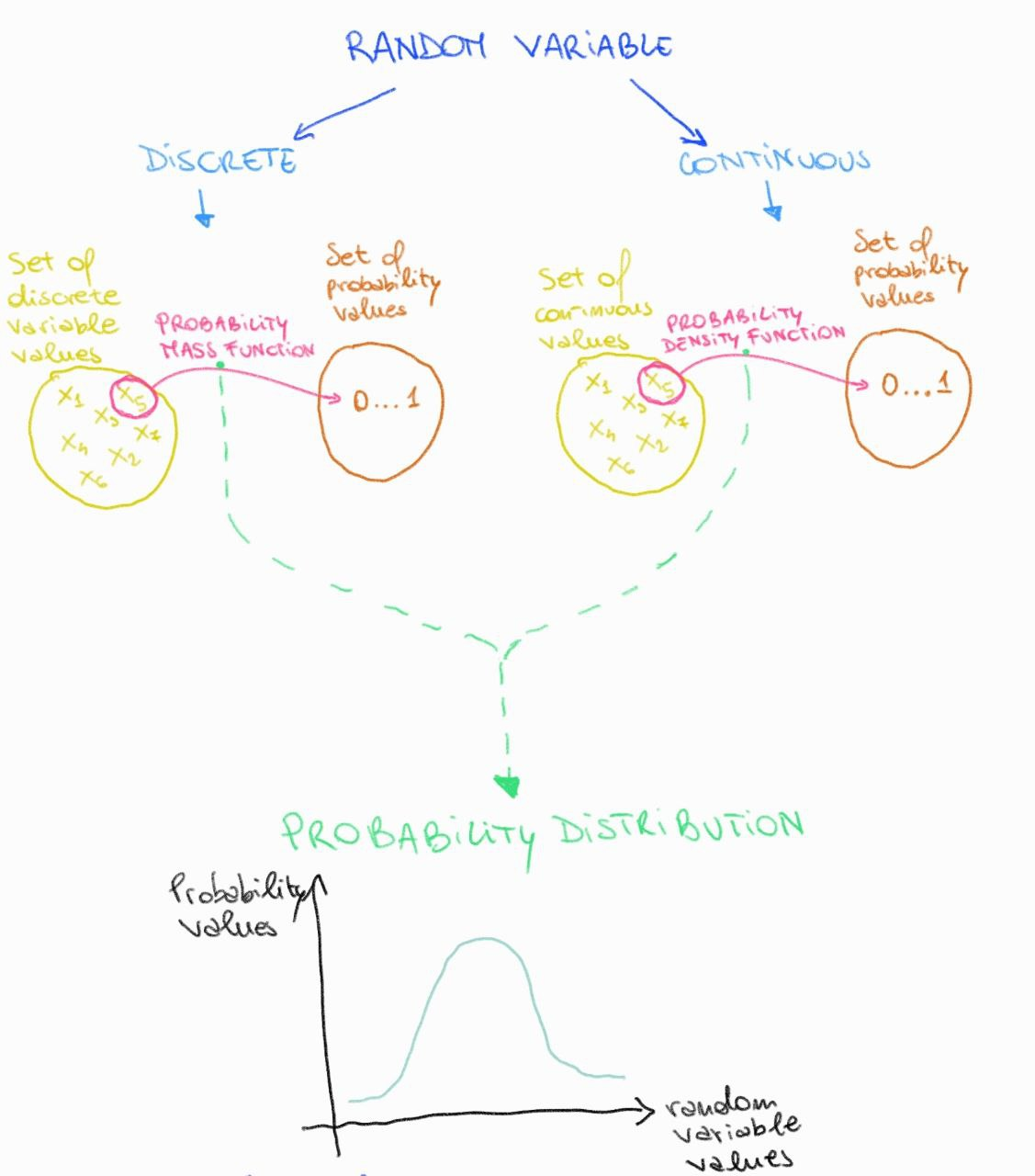Houston, we have a problem.

As you may have noticed, each random variable possesses a specific probability mass function or probability density function that connects its values with their respective probabilities. Consequently, every random variable has a specific distribution of relative probability values.

Thus, it's easy to understand that, since random variables are infinite, the types of probability functions and the types of probability distribution graphs are also infinite.

So, every time you encounter a new random variable that no one has studied before and you want to define the distribution of relative probability values, you have to mathematically derive the complex and detailed probability function that allows you to link the variable's values to probability values. Good luck with that. I dare say you find yourself in a rather challenging situation.Are you still there? Has someone hidden under the bed?

Take courage, there's good news in all of this. Over the past 400 years, statisticians have studied numerous random variables and have derived their probability functions, thus also obtaining the distributions of relative probability values. In short, they have put in a significant effort to simplify our lives. From this extensive effort, it has become apparent that there are probability functions that adapt to different types of random variables, and for this reason, they are called notable functions. Of course, the respective distributions of probability values are defined as notable distributions.

I tried to describe the concept of notable probability functions in various ways and, in the end, I found this solution by observing my three-year-old niece. Two days ago, I handed her an orange, and she exclaimed, "What a beautiful ball!" Her statement was an approximation. We have several balls of that size at home, perfectly spherical and colored. Therefore, she used the ball as a model, meaning she adapted the concept of a ball to the orange because she adhered to criteria of shape and dimensions that are usually attributed to the balls she typically plays with.

Perhaps this example is a bit convoluted, but I hope you can grasp the analogy. When facing a random variable that adheres to criteria akin to probability functions and notable distributions, we can serenely use this as a model and transfer the relevant information about the variable in question, without needing to derive the probability or density function from scratch, which would require significant mathematical effort.

So, when dealing with a random variable, don't despair. Try to understand the characteristics of it and the experiment (or event) in question. Based on the criteria being met, apply a notable probability function (as well as the corresponding notable distribution), and you can easily deduce the probability of observing a certain value for that variable. Fascinating, isn't it?

I bet you're wondering what these notable distributions are. Well, there are many of them. Describing them all is exhaustive and rather unnecessary, given that a simple web search can provide the criteria and applications of a specific notable distribution. But to better understand their use, I've decided to present some of the most well-known ones below, along with examples and a bit of code. So, take a short break, have a coffee, and let's get started.

THE BINOMIAL DISTRIBUTION

• Described towards the end of the seventeenth century by the Swiss mathematician Jean Bernoulli (hence referred to as the Bernoulli distribution).
• It is a distribution of probability values related to discrete random variables.
-Its probability function is used to calculate the probability of obtaining a certain number of successes following a certain number of repetitions of the same experiment.
• The criteria that must be met to apply this distribution to a certain random variable are:
a) Each trial conducted must have only two possible and incompatible outcomes. They are generally referred to as success and failure.
b) Each trial of the experiment must be independent of all others. Thus, the fact that one trial has resulted in failure does not influence the occurrence of success or failure in subsequent trials.
c) The probability of obtaining a success (p) must be the same in all trials and therefore constant, as is the probability of obtaining a failure (q = 1 - p).
• The formula for the probability function concerning the binomial distribution is as follows:Now let's look at an example: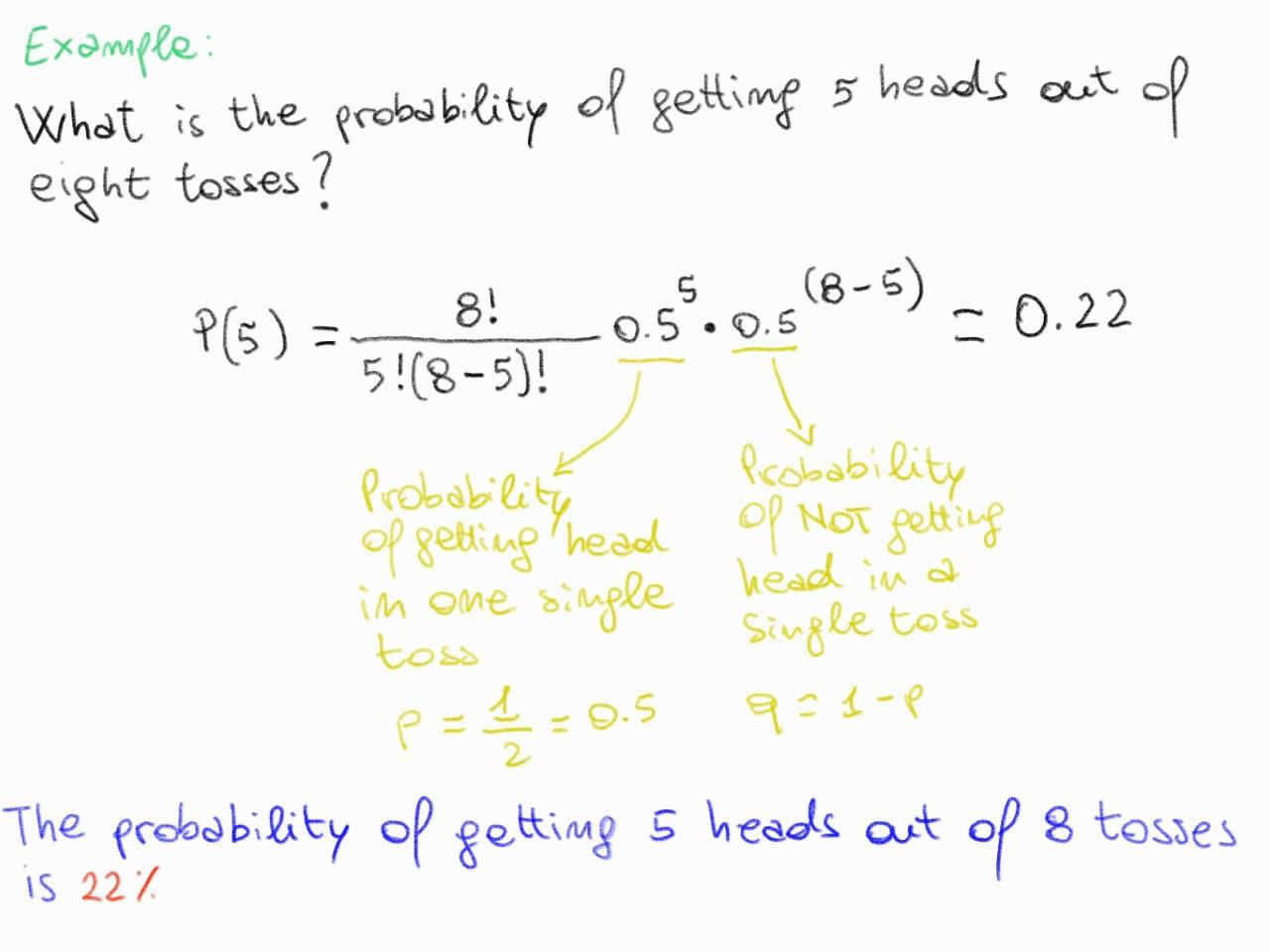THE POISSON DISTRIBUTION

• Described in the early nineteenth century by the Frenchman Simeon-Denis Poisson.
• It is a distribution of probability values related to discrete random variables.
• Its probability function is used to calculate the probability of obtaining a certain number of successes per unit of time or, in general, within a continuous interval (such as an area or length unit).
• The criteria that must be met to apply this distribution to a certain random variable are:
a) Events must be independent.
b) The number of times a success occurs in a unit of time must be independent of the number of observed successes in other time units. For example, the fact that 5 successes were observed at time x does not influence the number of successes observed at time y.
c) The average number of successes per unit of time must be constant.
• The formula for the probability function concerning the Poisson distribution is as follows:Now let's look at an example:THE NORMAL DISTRIBUTION

• Described about two centuries ago by the German mathematician Karl Friedrich Gauss, the normal distribution is a probability distribution of values for continuous random variables, characterized by a classic bell-shaped curve.
• It is a widely used distribution as it can describe the probabilities of values for continuous random variables in many natural, biological, medical, economic, physical, and other phenomena, where values close to the mean are the most probable, while those at the extremes are less likely. Therefore, in this distribution, a bell-shaped curve is observed with probability values symmetric around the mean of the random variable. Furthermore, the mean value coincides with the mode and the median.
• The criteria that must be satisfied to apply this distribution to a certain random variable are:
a) The mean, median, and mode must coincide.
b) Probability values are symmetric with respect to the mean.
• The formula for the probability density function of the normal distribution is as follows:It is evident that the normal probability distribution is determined by the mean and the standard deviation of the random variable under consideration. As these parameters vary, a bell-shaped curve of different heights and widths is observed, while maintaining symmetry of probability values around the mean. Please observe the image below to understand how different bell-shaped curve types of probability distribution are obtained by varying the random variable and thus, the mean and standard deviation.From the image above, it can also be noticed how complex it is to compare different normal probability distributions. However, there is a solution in this case as well. If we want to compare two or more normal probability distributions, we can convert them into a standard version known as the standard normal distribution, where the mean is 0 and the standard deviation is 1.

You might be wondering how it's possible to standardize a normal distribution.

You need to know that any normal distribution can be converted into its standard version simply by setting the mean to 0 and the standard deviation to 1, and calculating the standardized value of each value of the continuous random variable using the following formula:As mentioned earlier, bringing two normal distributions to standard conditions allows us to compare the variables in question better. If this is still not clear, observe the example provided below.

Standardization Example:

University student Ivar has taken two exams. In the first exam, the score is out of 30 and Ivar scored 26/30, while in the second exam, the score is out of 100 and Ivar scored 76/100. We also know that the average score for the first exam is 23.5/30 with a standard deviation of 2. Similarly, the average score for the second exam is 69/100 with a standard deviation of 6. At this point, we can ask in which exam Ivar performed better.

Directly comparing the values of the two exams is difficult as they are on different scales. Even comparing the normal distribution of exam 1 (mean = 23.5/30 and sd = 2) with the normal distribution of exam 2 (mean = 69/100 and sd = 6) to answer the question may not be helpful, as can be seen from the graph below obtained using R code.

``````# Creating data for both normal curves
### Exam 1
mean1 <- 23.5
standard_deviation1 <- 2
### Exam 2
mean2 <- 69
standard_deviation2 <- 6

# Not standard value of variable exam results
exam1_values <- seq(min(mean1 - 4 * standard_deviation1),
max(mean1 + 4 * standard_deviation1), length = 1000)

exam2_values <- seq(min(mean2 - 4 * standard_deviation2),
max(mean2 + 4 * standard_deviation2), length = 1000)

# build a normal distribution curve using the normal probability density function
probability_density_not_standardized_exam1 <- dnorm(exam1_values, mean = mean1, sd = standard_deviation1)
probability_density_not_standardized_exam2 <- dnorm(exam2_values, mean = mean2, sd = standard_deviation2)

# Creating the plot with the two overlaid curves
par(mfrow = c(1, 2))
p1 <- plot(exam1_values, probability_density_not_standardized_exam1, type = "l", xlab = "Exam1 Values", ylab = "Probability Density",
main = "Normal Probability Curve exam 1")
p2 <- plot(exam2_values, probability_density_not_standardized_exam2, type = "l", xlab = "Exam2 Values", ylab = "Probability Density",
main = "Normal Probability Curve exam 2")
par(mfrow = c(1, 1))``````So, using non-standard values, it is complex to understand in which exam Ivar has achieved better results. Even more challenging is doing so by comparing the means and standard deviations of the non-standard values. At this point, let's proceed to obtain the standard values for both variables under examination (exam grade 1 and exam grade 2), in order to be able to determine in which exam Ivar has performed better.

``````# let's standardize all exams values
z_values_exam1 <- (exam1_values - mean1) / standard_deviation1
z_values_exam2 <- (exam2_values - mean2) / standard_deviation2

probability_density_standardized_exam1 <- dnorm(z_values_exam1, mean = 0, sd = 1)
probability_density_standardized_exam2 <- dnorm(z_values_exam2, mean = 0, sd = 1)

# Creating the plot with the two overlaid curves
par(mfrow = c(1, 2))
plot(z_values_exam1, probability_density_standardized_exam1, type = "l", xlab = "Exam1 standardized Values", ylab = "Probability Density",
main = "Normal Probability Curve exam 1")
plot(z_values_exam2, probability_density_standardized_exam2, type = "l", xlab = "Exam2 standardized Values", ylab = "Probability Density",
main = "Normal Probability Curve exam 2")
par(mfrow = c(1, 1))

# Now that both distributions have been standardized, we can compare the standard values of the two variables,
# grade for exam 1 and grade for exam 2, in order to understand in which exam Ivar performed better.Running the code provided above, it can be observed that Ivar's standardized score for exam 1 is 1.25, while that for exam 2 is 1.17. Now that the grades are on the same scale and thus easily comparable, we can state that Ivar scored higher on the first exam and therefore performed better compared to the second one.

I hope by this point you have understood the importance of standardization and the standard normal distribution. If not, feel free to write your questions in the comments below, and we will discuss any doubts together.

Before concluding this article, I would like to provide two important clarifications.

Shape indices of a distribution

There are two indices, Skewness and Kurtosis, that allow describing the shape of a distribution.

Skewness:

Skewness is a measure of the shape of a data distribution. It indicates how much a distribution deviates from symmetry. A symmetric distribution, like the standard normal distribution, will have a skewness of zero, which means that the tails of the distribution (the tails are the extreme parts of the distribution) are balanced around the mean. When skewness is different from zero, the distribution is asymmetric.

• A positive skewness indicates that the long tail of the distribution is oriented towards the right (to the right of the mean), and the distribution tends to concentrate on the left side.
• A negative skewness indicates that the long tail of the distribution is oriented towards the left (to the left of the mean), and the distribution tends to concentrate on the right side.
In general, a higher absolute skewness indicates a greater deviation from symmetry. Skewness is useful for understanding how data is distributed relative to its mean.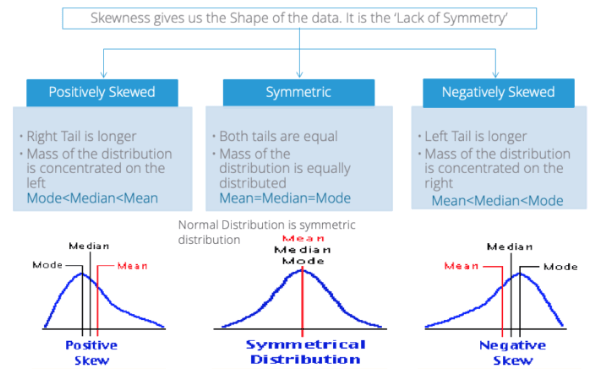It's possible calculate the skewness in R:

``````# Creating data for both normal curves
### Exam 1
mean1 <- 23.5
standard_deviation1 <- 2
### Exam 2
mean2 <- 69
standard_deviation2 <- 6
# Not standard value of variable exam results
exam1_values <- seq(min(mean1 - 4 * standard_deviation1),
max(mean1 + 4 * standard_deviation1), length = 1000)
exam2_values <- seq(min(mean2 - 4 * standard_deviation2),
max(mean2 + 4 * standard_deviation2), length = 1000)
# build a normal distribution curve using the normal probability density function
probability_density_not_standardized_exam1 <- dnorm(exam1_values, mean = mean1, sd = standard_deviation1)
probability_density_not_standardized_exam2 <- dnorm(exam2_values, mean = mean2, sd = standard_deviation2)
# calculate skewness
moments::skewness(probability_density_not_normalized_exam1)
moments::skewness(probability_density_not_normalized_exam2)``````

Kurtosis (Curtosis):

Kurtosis is a statistical measure that describes the shape, or more specifically, the "tailedness" of a probability distribution. It provides information about the concentration of data in the tails of a distribution compared to a normal distribution (also known as the Gaussian distribution). Remember that "tails" are the tapering ends on either side of a distribution. They represent the probability or frequency of values that are extremely high or low compared to the mean.

There are three main types of kurtosis:

• Leptokurtic Distribution: A distribution with positive kurtosis is called leptokurtic. In a leptokurtic distribution, the tails of the distribution are heavier (contain more extreme values) than those of a normal distribution. This indicates that the data has more values in the tails and fewer in the central part of the distribution. This is often associated with a higher probability of extreme events.

• Mesokurtic Distribution: A distribution with zero kurtosis is referred to as mesokurtic. A mesokurtic distribution has tails similar to a normal distribution. It means that the distribution doesn't have an unusual amount of values in its tails, and its shape resembles that of a normal distribution.

• Platykurtic Distribution: A distribution with negative kurtosis is called platykurtic. In a platykurtic distribution, the tails are lighter (contain fewer extreme values) than those of a normal distribution. This indicates that the data has fewer values in the tails and more in the central part of the distribution. This often results in a flatter peak compared to a normal distribution.As for the skewness is possible to calculate the kurtosis in R:

``````# Creating data for both normal curves
### Exam 1
mean1 <- 23.5
standard_deviation1 <- 2
### Exam 2
mean2 <- 69
standard_deviation2 <- 6
# Not standard value of variable exam results
exam1_values <- seq(min(mean1 - 4 * standard_deviation1),
max(mean1 + 4 * standard_deviation1), length = 1000)
exam2_values <- seq(min(mean2 - 4 * standard_deviation2),
max(mean2 + 4 * standard_deviation2), length = 1000)
# build a normal distribution curve using the normal probability density function
probability_density_not_standardized_exam1 <- dnorm(exam1_values, mean = mean1, sd = standard_deviation1)
probability_density_not_standardized_exam2 <- dnorm(exam2_values, mean = mean2, sd = standard_deviation2)
# calculate kurtosis
moments::kurtosis(probability_density_not_normalized_exam1)
moments::kurtosis(probability_density_not_normalized_exam2)``````

Both skewness and kurtosis provide information about the shape of the data distribution and can be used to better understand the characteristics of data in a statistical context.

How can we determine which probability distribution the variable under examination belongs to?

In my work as a bioinformatician, I often find myself verifying which probability distribution the variable under examination belongs to. To understand this, I make use of the Quantile-Quantile (Q-Q) plot.

In particular, the QQ plot is a graph used to assess whether a data distribution is similar to a theoretical distribution (typically the normal distribution). In practice, a QQ plot compares the observed quantiles of the data with the expected quantiles from the theoretical distribution. If the points in the QQ plot roughly follow a diagonal line, it means that the data follows the theoretical distribution. Deviations from the diagonal line suggest that the data may not follow the theoretical distribution. (Take a look at the code in R below)

``````# Generate random data from a normal distribution
set.seed(123)  # For reproducibility
data <- rnorm(100)

# Create the QQ plot
qqnorm(data, main = "QQ Plot")
qqline(data, col = "red")``````Furthermore, the Q-Q plot also allows for the comparison of two probability distributions in order to understand whether they are similar or different.

In any case, I urge you to watch the video provided below in which the dear and talented Josh Starmer explains this concept in detail.

Well, we've reached the end of this article, and thus we have concluded the chapter on this other branch of statistics, namely the theory of probability. I want to reiterate that in this article, we have only discussed some of the probability distributions related to the values of a random variable. There are many other equally important distributions, as you can see from these two excellent articles: link1 e link2.
Regarding this series on statistics, I'll see you in the next article where we will start discussing inferential statistics, the final branch of statistics.

So, see you soon. Keep following, commenting, and participating in this informative journey.

P.S. This is a brief summary outline of probability theory:Resources:

Omar Almolla